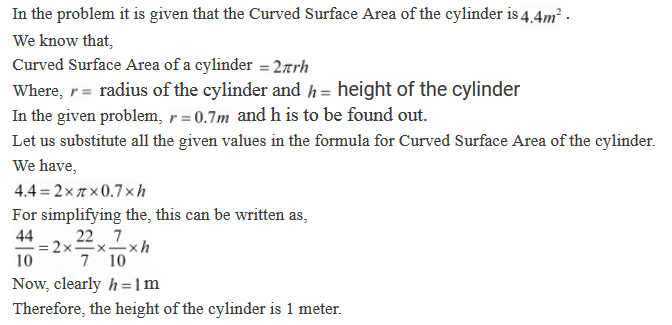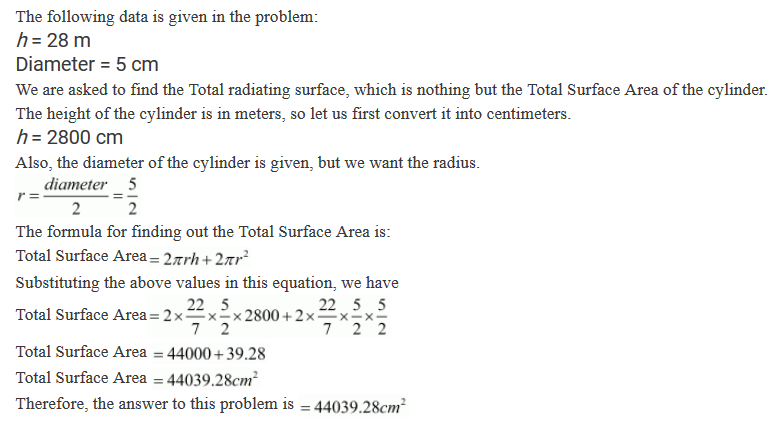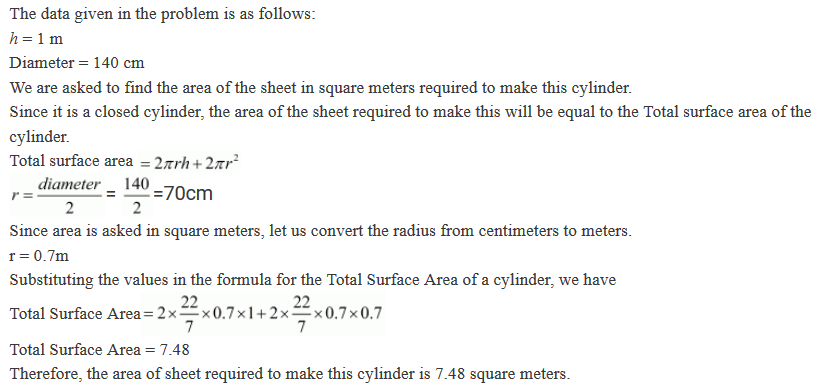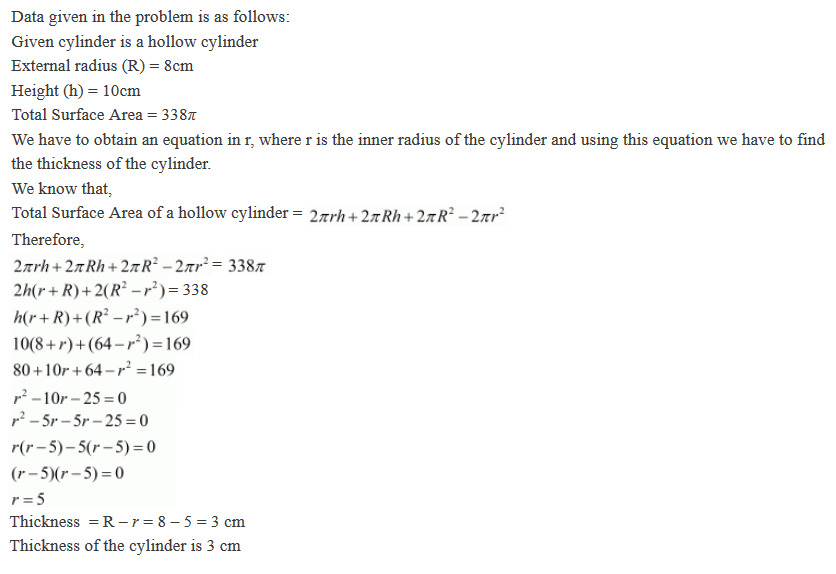#### Chapter 19 Surface Area and Volume of a Right Circular Cylinder R.D. Sharma Solutions for Class 9th Exercise 19.1

Exercise 19.1

1. Curved surface area of a right circular cylinder is 4.4 m2. If the radius of the base of the cylinder is 0.7 m, find its height.

Solution2. In a hot water heating system, there is a cylindrical pipe of length 28 m and diameter 5 cm. Find the total radiating surface in the system.

Solution3. A cylindrical pillar is 50 cm in diameter and 3.5 m in height. Find the cost of painting the curved surface of the pillar at the rate of 12.50 per m2.

Solution4. It is required to make a closed cylindrical tank of height 1 m and base diameter 140 cm from a metal sheet. How many square metres of the sheet are required for the same?

Solution5. A solid cylinder has total surface area of 462 cm2. Its curved surface area is one-third of its total surface area. find the radius and height of the cylinder.

Solution6. The total surface area of a hollow cylinder which is open from both sides is 4620 sq. cm, area of base ring is 115.5 sq. cm and height 7 cm. Find the thickness of the cylinder.

Solution7. Find the ratio between the total surface area of a cylinder to its curved surface area, given that its height and radius are 7.5 cm and 3.5 cm.

Solution8. The total surface area of a hollow metal cylinder, open at both ends of external radius 8 cm and height 10 cm is 338 p cm2. Taking r to be inner radius, obtain an equation in r and use it to obtain the thickness of the metal in the cylinder.

Solution9. A cylindrical vessel, without lid, has to be tin-coated on its both sides. If the radius of the base is 70 cm and its height is 1.4 m, calculate the cost of tin-coating at the rate of Rs 3.50 per 1000 cm2.

Solution10. The inner diameter of a circular well is 3.5 m. It is 10 m deep Find:
(i) inner curved surface area.
(ii) the cost of plastering this curved surface at the rate of Rs 40 per m2

Solution11. Find the lateral curved surface area of a cylinderical petrol storage tank that is 4.2 m in diameter and 4.5 m high. How much steel was actually used, if 1/12 of steel actually used was wasted in making the closed tank?

Solution12. The students of a Vidyalaya were asked to participate in a competition for making and decorating pen holders in the shape of a cylinder with a base, using cardboard. Each pen holder was to be of radius 3 cm and height 10.5 cm. The Vidyalaya was to supply the competitors with carboard. If there were 35 competitors, how much cardboard was required to be bought for the competition?

Solution13. The diameter of roller 1.5 m long is 84 cm. If it takes 100 revolutions to level a playground, find the cost of levelling this ground at the rate of 50 paise per square metre.

Solution14.Twenty cylindrical pillars of the Parliament House are to be cleaned. If the diameter of each pillar is 0.50 m and height is 4 m. What will be the cost of cleaning them at the rate of Rs 2.50 per square metre?

Solution